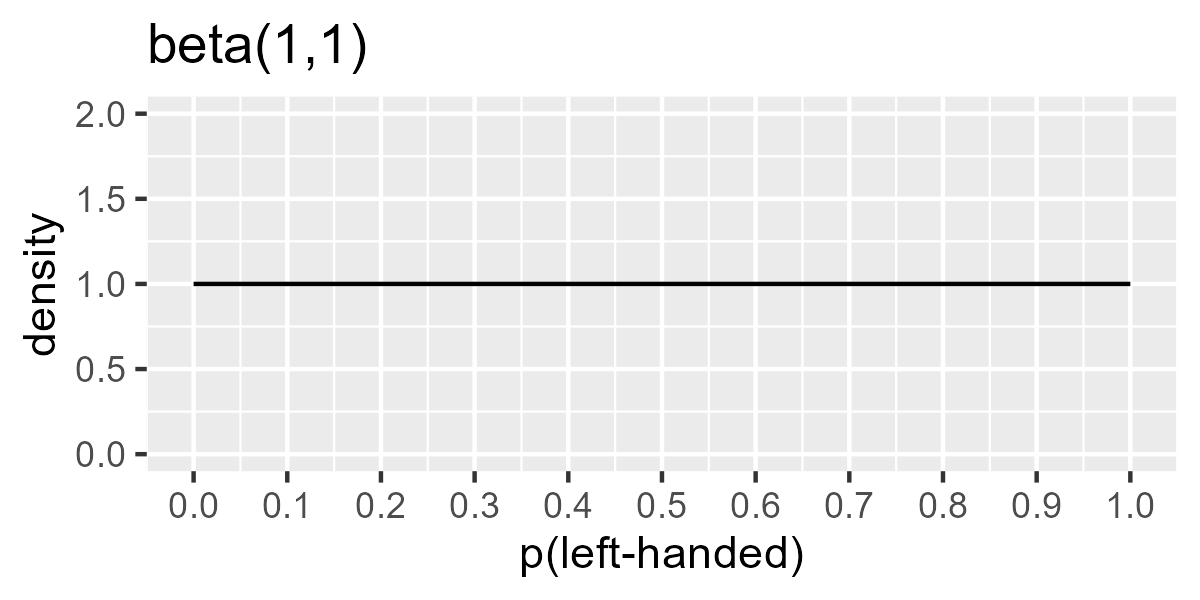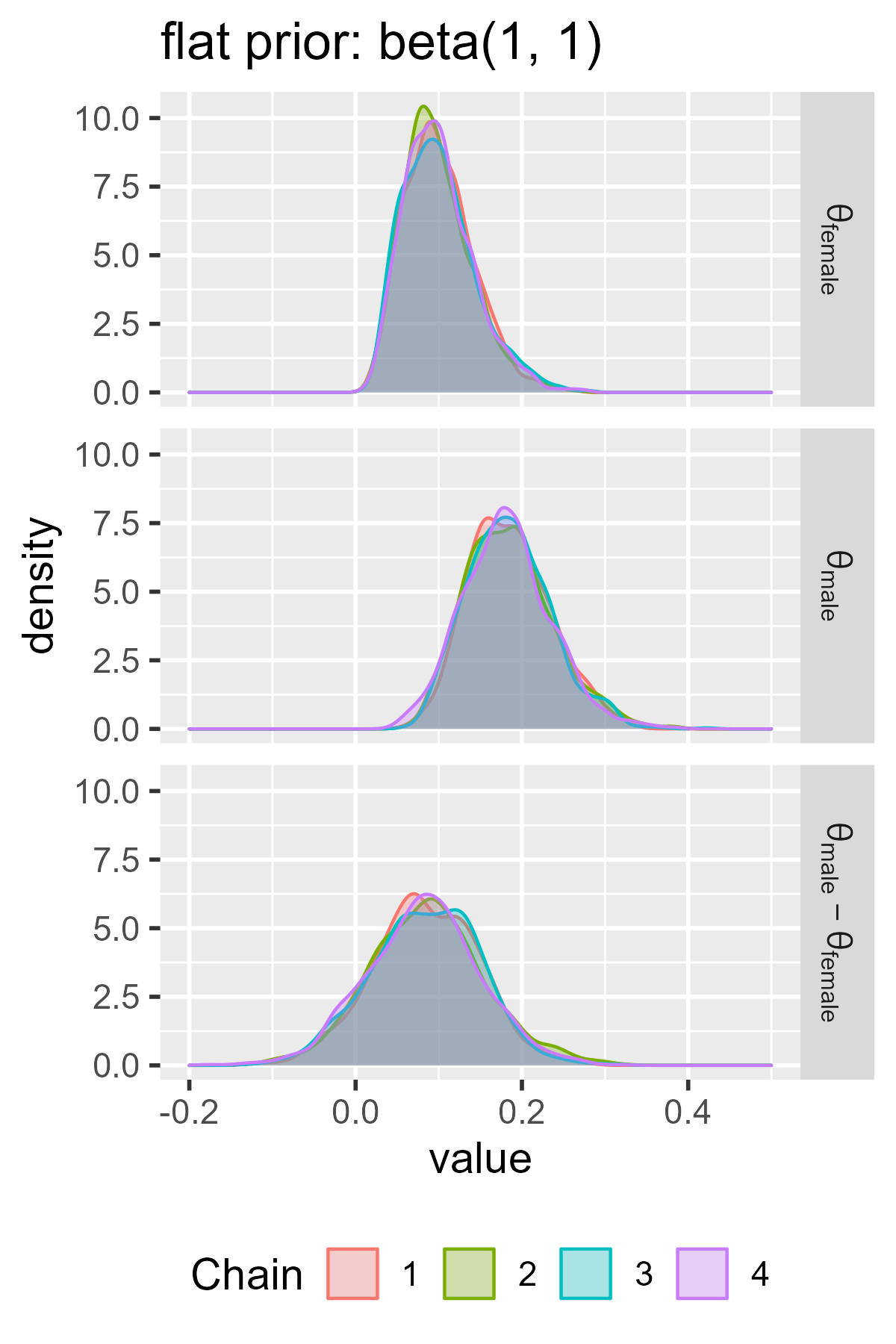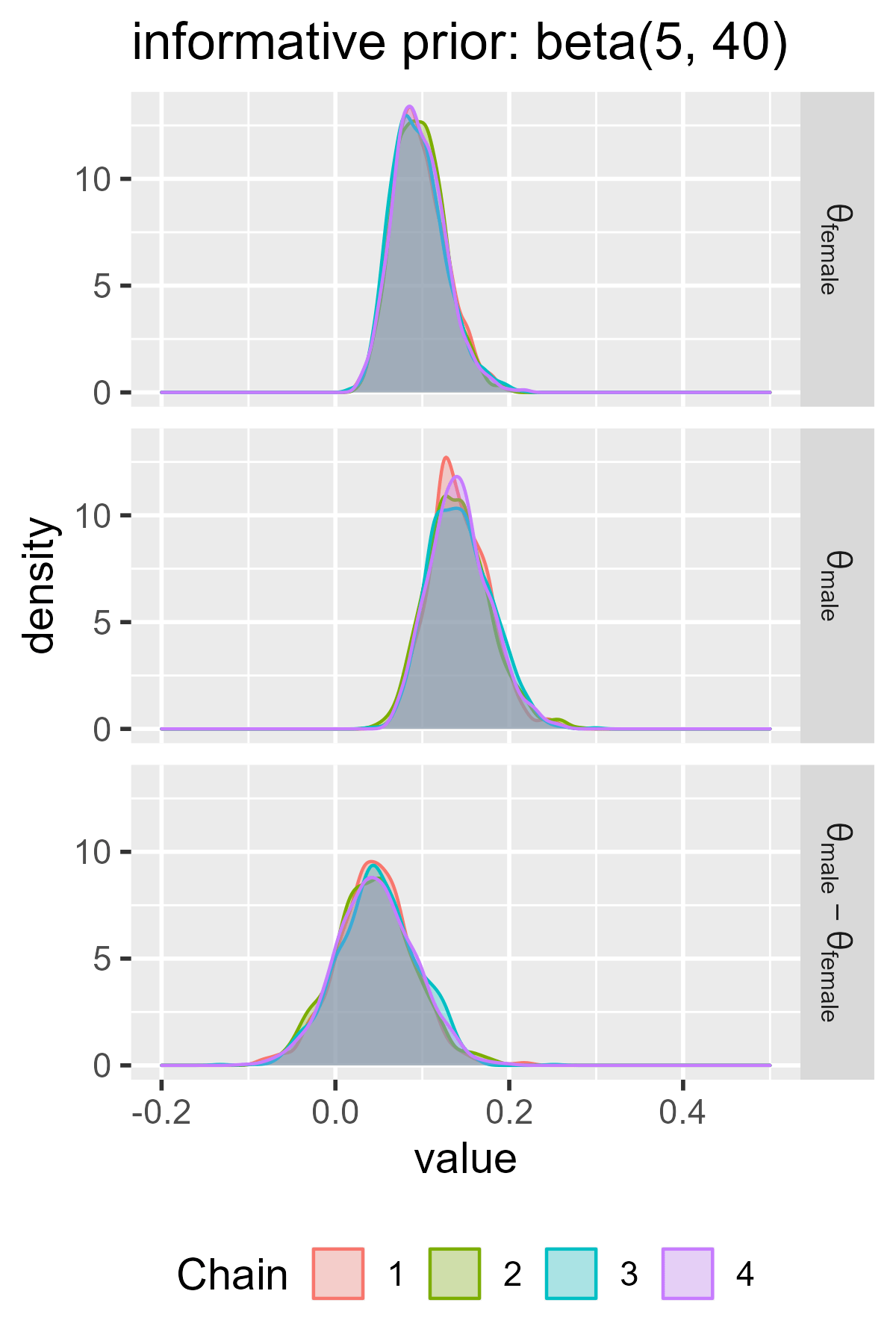# I don’t know Fisher’s exact test, but I know Stan

May 15, 2017
By

Want to share your content on R-bloggers? click here if you have a blog, or here if you don't.

A few days ago, I watched a terrific lecture by Bob Carpenter on
Bayesian models. He started with a Bayesian approach to Fisher’s exact test. I
had never heard of this classical procedure, so I was curious to play with the
example. In this post, I use the same data that he used in the lecture and
in an earlier, pre-Stan blog post. I show how I would go about
fitting the model in Stan and inspecting the results in R.

## Problem statement

We observed the following data.

sex n left handed n right handed
male 9 43
female 4 44

Question: Is the rate of left-handedness different between the male and female
groups? Specifically, is left-handedness more likely in the male group?

### Classical approach

In frequentist statistics, we might run Fisher’s exact test. At least, that’s what
the flow charts tell us. To do that, we first put the data in a
matrix.

# Create a matrix representation of the data for the fisher test
m <- matrix(c(9, 43, 4, 44), nrow = 2, byrow = TRUE,
dimnames = list(sex = c("male", "female"),
handedness = c("left", "right")))
m
#>         handedness
#> sex      left right
#>   male      9    43
#>   female    4    44


We can run the two-tailed test: Are the two groups different?

fisher.test(m)
#>
#> 	Fisher's Exact Test for Count Data
#>
#> data:  m
#> p-value = 0.2392
#> alternative hypothesis: true odds ratio is not equal to 1
#> 95 percent confidence interval:
#>   0.582996 10.927993
#> sample estimates:
#> odds ratio
#>   2.283832


The output is a little verbose, but I do like how it spells out a sentence
describing the alternative hypothesis.

We can also consider one-sided test: Is left-handedness greater in the male
group?

fisher.test(m, alternative = "greater")
#>
#> 	Fisher's Exact Test for Count Data
#>
#> data:  m
#> p-value = 0.1502
#> alternative hypothesis: true odds ratio is greater than 1
#> 95 percent confidence interval:
#>  0.7006563       Inf
#> sample estimates:
#> odds ratio
#>   2.283832


In both tests, we cannot reject the null hypothesis because the p-value is
greater than .05 :x:, so we would conclude that the two groups are not different.

But I don’t really know this test that well. We never covered it in any of my
stats classes, and indeed, this post is the first time I ever used the function
fisher.test(). If I had never heard of the test, I am not quite sure what I
would have done. Maybe a logistic regression (p = .191 :x:).

## Creating a Stan model

I’m very not fluent in the classical bag of tricks, but that’s okay. I know some
Stan, I have an idea about how the data could have been generated, and that’s
good enough :relaxed:. I can just write down my data-generating story in a model
and let Stan compute a posterior distribution for the difference in handedness
rates between the two groups.

For my model, I’m going to suppose that in each group, there is a probability of
being left-handed called θ and that the counts we see result from a
binomial process. The 9 left-handed males we observe are the number of successes
from 52 observations of a process that “succeeds” with probability
θmale.

To fit the model, we need a prior distribution. The prior’s job is to generate
possible θ’s, and we will use our data to update the prior. The Beta
distribution generates values between 0 and 1, so it’s an obvious choice.

In math, the model for a group would be:

We can use a flat, uninformative prior by using a = 1, b = 1. This
prior considers all probabilities from 0 to 1 as equally plausible.

library(ggplot2)
steps <- seq(from = 0, to = 1, by = .01)

ggplot(data.frame(x = steps, y = dbeta(steps, shape1 = 1, shape2 = 1))) +
geom_line(aes(x, y)) +
scale_x_continuous(breaks = (0:10) / 10) +
ylim(0, 2) +
labs(x = "p(left-handed)", y = "density", title = "beta(1,1)")But I also think that 10-ish% of people are left handed. (I don’t know where I
first heard this number, but it’ll serve as my prior information.) I toyed
around with shape1 and shape2 parameters in dbeta() until I got the prior
Beta(5, 40), which is peaked around .1-ish but wide enough to keep .5 and .15 as
plausible values too.

ggplot(data.frame(x = steps, y = dbeta(steps, shape1 = 5, shape2 = 40))) +
geom_line(aes(x, y)) +
scale_x_continuous(breaks = (0:10) / 10) +
labs(x = "p(left-handed)", y = "density", title = "beta(5,40)")Let’s write out a really simple model in Stan. Okay, it used to be really
simple. Then I made the parameters for the Beta prior data values, and then I
created an option to just sample the prior distribution. But the core of it is
simple. The most important lines are the one with ~ symbols. These
correspond to the sampling statements in the mathematical description of the
model.

model_code <- "
data {
int beta_a;
int beta_b;
int n_total_1;
int n_total_2;
int n_hits_1;
int n_hits_2;
int sample_prior_only;
}
parameters {
real theta_1;
real theta_2;
}
model {
theta_1 ~ beta(beta_a, beta_b);
theta_2 ~ beta(beta_a, beta_b);

if (sample_prior_only != 1) {
n_hits_1 ~ binomial(n_total_1, theta_1);
n_hits_2 ~ binomial(n_total_2, theta_2);
}
}
generated quantities {
real diff;
diff = theta_1 - theta_2;
}
"


The generated quantities block runs on every sample of the posterior
distribution. Here, we compute the
θmale − θfemale on every
draw. Computing the difference inside the model code means that Stan
will treat the diff values like any other parameter of the model. It will show
up in summary functions and in plots of model parameters. That saves us some
work later on.

library(rstan)
#> rstan (Version 2.15.1, packaged: 2017-04-19 05:03:57 UTC, GitRev: 2e1f913d3ca3)
#> For execution on a local, multicore CPU with excess RAM we recommend calling
#> rstan_options(auto_write = TRUE)
#> options(mc.cores = parallel::detectCores())


I begin by compiling the model. This step will create an executable
program that can sample from the model. I do the compilation in its own step so
that I can re-use the program for different versions of the model.

model_program <- stan_model(model_code = model_code)


For convenience, I wrote a function that fits different versions of this model.
This step is not necessary, but I don’t like repeating myself. (Normally, you
would use sampling(model_program, stan_data) to get samples from a
model-program.)

run_model <- function(beta_a, beta_b, sample_prior_only) {
stan_data <- list(
beta_a = beta_a,
beta_b = beta_b,
n_total_1 = 9 + 43,
n_total_2 = 4 + 44,
n_hits_1 = 9,
n_hits_2 = 4,
sample_prior_only = sample_prior_only)

# Use quietly() to hide the sampler's output text
model <- purrr::quietly(sampling)(model_program, stan_data)

# But print any warnings that would have appeared
invisible(lapply(model$warnings, warning, call. = FALSE)) model$result
}


The actual left-handed versus right-handed numbers are hard-coded, but I can
adjust the parameters for the Beta prior and toggle between sampling from the
prior and the posterior. Stan normally prints out verbose progress information
but I suppress that by using purrr::quietly(). I still want warnings, so I
print them if they arise.

### Checking our prior information

Now, let’s draw samples from the priors of each model. This step lets us check
that our program works as expected. We know how the values should be

m_informative_pd <- run_model(beta_a = 5, beta_b = 40, sample_prior_only = 1)
m_informative_pd
#> Inference for Stan model: 7e241131e72a0ec0b2cfd4f9a73290f1.
#> 4 chains, each with iter=2000; warmup=1000; thin=1;
#> post-warmup draws per chain=1000, total post-warmup draws=4000.
#>
#>           mean se_mean   sd   2.5%    25%    50%    75%  97.5% n_eff Rhat
#> theta_1   0.11    0.00 0.05   0.04   0.08   0.11   0.14   0.22  3047    1
#> theta_2   0.11    0.00 0.05   0.04   0.08   0.11   0.14   0.21  2953    1
#> diff      0.00    0.00 0.06  -0.13  -0.04   0.00   0.04   0.13  3072    1
#> lp__    -32.40    0.02 0.99 -35.01 -32.81 -32.11 -31.68 -31.42  1776    1
#>
#> Samples were drawn using NUTS(diag_e) at Tue May 16 14:05:44 2017.
#> For each parameter, n_eff is a crude measure of effective sample size,
#> and Rhat is the potential scale reduction factor on split chains (at
#> convergence, Rhat=1).

m_flat_pd <- run_model(beta_a = 1, beta_b = 1, sample_prior_only = 1)
m_flat_pd
#> Inference for Stan model: 7e241131e72a0ec0b2cfd4f9a73290f1.
#> 4 chains, each with iter=2000; warmup=1000; thin=1;
#> post-warmup draws per chain=1000, total post-warmup draws=4000.
#>
#>          mean se_mean   sd  2.5%   25%   50%   75% 97.5% n_eff Rhat
#> theta_1  0.49    0.01 0.28  0.03  0.25  0.49  0.74  0.97  3134    1
#> theta_2  0.50    0.00 0.28  0.03  0.26  0.51  0.74  0.97  3247    1
#> diff    -0.01    0.01 0.40 -0.77 -0.29 -0.01  0.28  0.75  3001    1
#> lp__    -3.90    0.03 1.09 -6.86 -4.34 -3.56 -3.12 -2.81  1362    1
#>
#> Samples were drawn using NUTS(diag_e) at Tue May 16 14:05:45 2017.
#> For each parameter, n_eff is a crude measure of effective sample size,
#> and Rhat is the potential scale reduction factor on split chains (at
#> convergence, Rhat=1).


We can confirm (by inspecting the diff row) that the difference in
left-handedness in both groups is 0 according to our priors. The
informative prior says that the values between 0.04 and
0.22 are plausible rates of left-handedness in each group.

It’s worth a moment to reflect on how obviously wrong the uninformative prior is.
The central θ value in each group is .5. Therefore, half of the prior
samples assert that there are more left-handed individuals than right-handed
ones! If there is anything we know about handedness, it’s that left-handedness is
less common than right-handedness. Uninformative sometimes connotes “unbiased”
or “letting the data speak for itself”, but in this case, I would say
“gullible”.

## Sampling the posterior

Now, the fun part. We update our prior information with our data.

m_informative <- run_model(beta_a = 5, beta_b = 40, sample_prior_only = 0)
m_informative
#> Inference for Stan model: 7e241131e72a0ec0b2cfd4f9a73290f1.
#> 4 chains, each with iter=2000; warmup=1000; thin=1;
#> post-warmup draws per chain=1000, total post-warmup draws=4000.
#>
#>           mean se_mean   sd   2.5%    25%    50%    75%  97.5% n_eff Rhat
#> theta_1   0.14    0.00 0.04   0.08   0.12   0.14   0.17   0.22  3288    1
#> theta_2   0.10    0.00 0.03   0.05   0.07   0.09   0.11   0.17  2837    1
#> diff      0.05    0.00 0.05  -0.05   0.02   0.05   0.08   0.14  3020    1
#> lp__    -70.65    0.02 1.05 -73.56 -71.09 -70.33 -69.89 -69.63  1834    1
#>
#> Samples were drawn using NUTS(diag_e) at Tue May 16 14:05:45 2017.
#> For each parameter, n_eff is a crude measure of effective sample size,
#> and Rhat is the potential scale reduction factor on split chains (at
#> convergence, Rhat=1).

m_flat <- run_model(beta_a = 1, beta_b = 1, sample_prior_only = 0)
m_flat
#> Inference for Stan model: 7e241131e72a0ec0b2cfd4f9a73290f1.
#> 4 chains, each with iter=2000; warmup=1000; thin=1;
#> post-warmup draws per chain=1000, total post-warmup draws=4000.
#>
#>           mean se_mean   sd   2.5%    25%    50%    75%  97.5% n_eff Rhat
#> theta_1   0.19    0.00 0.05   0.09   0.15   0.18   0.22   0.30  2987    1
#> theta_2   0.10    0.00 0.04   0.04   0.07   0.10   0.13   0.20  2511    1
#> diff      0.09    0.00 0.07  -0.04   0.04   0.09   0.13   0.21  3042    1
#> lp__    -43.14    0.02 1.00 -45.82 -43.54 -42.84 -42.43 -42.15  1768    1
#>
#> Samples were drawn using NUTS(diag_e) at Tue May 16 14:05:46 2017.
#> For each parameter, n_eff is a crude measure of effective sample size,
#> and Rhat is the potential scale reduction factor on split chains (at
#> convergence, Rhat=1).


The flat model puts the difference at 0.09 and 90% of the plausible
values fall in the interval [-0.02, 0.19]. The informative
model is more skeptical of higher left-handedness rates, so it puts the difference
at 0.05 with 90% of the values between [-0.03, 0.13].
Both of these intervals contain 0 and negative values :x:, so there is not much
evidence for higher left-handedness in the male group.

To compute a “Bayesian p-value”, we could ask what proportion of differences
are 0 or negative. There are more proper ways to make this inference in a
Bayesian framework, but this approach is the easiest and it works for a model
this simple. If 10% of the plausible values for the group differences are
negative, then we assign a 10% probability to a negative group difference.

df_flat <- as.data.frame(m_flat)
mean(df_flat$diff <= 0) #>  0.09575 df_informative <- as.data.frame(m_informative) mean(df_informative$diff <= 0)
#>  0.15525


It’s also worth comparing the two models. I’ve recently become a fan of the
ggmcmc package for quick visualization
of Stan models. The package uses a function ggs() to create a long dataframe
of MCMC samples. Then you plug those dataframes into various plotting functions
that start with ggs_. I especially like how the package returns a plain
ggplot2 plot that I can easily adjust with a few extra lines of code.

For example, not much effort is required—after some practice and
trial-and-error, of course—to visualize the posterior samples in each model.

library(ggmcmc)
#>
#> Attaching package: 'tidyr'
#> The following object is masked from 'package:rstan':
#>
#>     extract

# A helper dataframe for relabeling parameters. I'm writing them in a way that
# works with ?plotmath conventions.
labels <- data.frame(
Parameter = c("theta_1", "theta_2", "diff"),
Label = c("theta[male]", "theta[female]", "theta[male] - theta[female]"))

# Get ggmcmc's tidy dataframe of each model.
# ggs() doesn't like that labels I made have brackets so I am suppressing its
# warnings.
ggs_flat <- suppressWarnings(
ggs(m_flat, description = "flat", par_labels = labels)
)
ggs_informative <- suppressWarnings(
ggs(m_informative, description = "informative", par_labels = labels)
)

ggs_density(ggs_flat) +
facet_grid(Parameter ~ ., labeller = label_parsed) +
ggtitle("flat prior: beta(1, 1)") +
theme_grey(base_size = 14) +
theme(legend.position = "bottom") +
# so the two models can be compared
xlim(-.2, .5)

ggs_density(ggs_informative) +
facet_grid(Parameter ~ ., labeller = label_parsed) +
ggtitle("informative prior: beta(5, 40)") +
theme_grey(base_size = 14) +
theme(legend.position = "bottom") +
# so the two models can be compared
xlim(-.2, .5)We can also compare the models together in a single plot by passing a list of
model dataframes into ggs_caterpillar().

ggs_caterpillar(D = list(ggs_flat, ggs_informative), line = 0,
thick_ci = c(0.05, 0.95), thin_ci = c(0.025, 0.975)) +
# Parse the labels as formatted math
scale_y_discrete(
breaks = as.character(unique(ggs_flat$Parameter)), labels = parse(text = as.character(unique(ggs_flat$Parameter)))) +
labs(caption = "Intervals: thick 90%, thin 95%. Point: median.",
y = NULL, x = NULL) +
theme_grey(base_size = 14)There’s a lot of useful information here. First, the intervals in the flat prior
model are wider than the ones for the informative model. The two models largely
agree on the values in the female group, although the flat prior model is wider.
These wider intervals indicate greater uncertainty about the parameter values.
The two models disagree on the male group, because the informative model assigns
little prior probability to values greater than .25 but the flat model doesn’t
discount those possibilities.

The models also demonstrate the regularizing effect of prior information.
Regularization broadly refers to techniques to avoid overfitting a dataset.
Priors can regularize a model by making it skeptical of certain parameter
values—in this case, high values of left-handedness. The male probabilities in
the flat model are basically pulled towards the values in the informative prior
(.1-ish). We can see this effect in how the midpoint is shifted in the flat
model versus the informative model.

I would be remiss if I didn’t end with the following disclaimer/trivia. In a
way, the question behind this post is ill-posed because handedness is not quite
a binary measure. Some years ago, I had a class on stuttering and fluency
disorders. (I used to be a speech pathologist.) There once was a lot of research
on the association between handedness and stuttering, and at some point,
researchers figured out that they could measure handedness as a continuous
measure. They gave people a survey asking which hands they use for certain tasks
and then computed a so-called dextrality quotient from the responses. I
had the concept driven home when I once saw my wife casually switch
between her left and right hands while brushing her teeth. I would probably poke
a hole through my cheek if I used my left hand! So: Handedness may be a matter
of degree. (Or maybe not. I mostly wanted to mention the dextrality quotient.

R-bloggers.com offers daily e-mail updates about R news and tutorials about learning R and many other topics. Click here if you're looking to post or find an R/data-science job.
Want to share your content on R-bloggers? click here if you have a blog, or here if you don't.